# AP Calculus AB Practice Test 49

### Test Information8 questions24 minutes

Calculator Allowed

1. Given that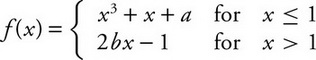, if fis differentiable at x= 1, what is the value of a+ 2b?

2. If g′(x) = cos(π)x2+ 1) and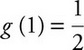, then g(2)=

3. The point (-2, 5) is on the graph of a differential function f, and f-2) = 3. What is the estimate for f(-1.9), using local linear approximation for fat x= -2?

4. If a function fhas its derivative f′(x) given as f′(x) = -3e-xcos x+ 2 for -2 < x< 2, on what interval(s) is fincreasing?

5. A particle moving in a straight line has a velocity of v(t) = 2-tcos (2t) feet per minute. What is the total distance in feet traveled by the particle when 0 ≤t≤ 3 minutes?

6. How many points of inflection does the graph of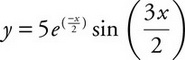have on the intervals (0, 2π)?

7. The table below shows selected values of a continuous function fon the closed interval [1,16].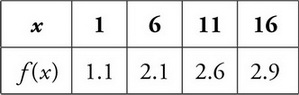Using three left endpoint rectangles of equal length (LRAM), what is the approximate value of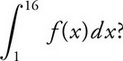8.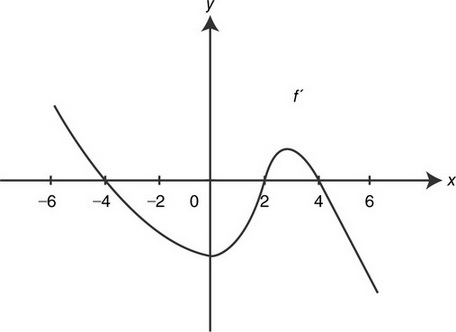The figure above shows the graph of the derivative of a function f. Which of the following could be the graph of f?